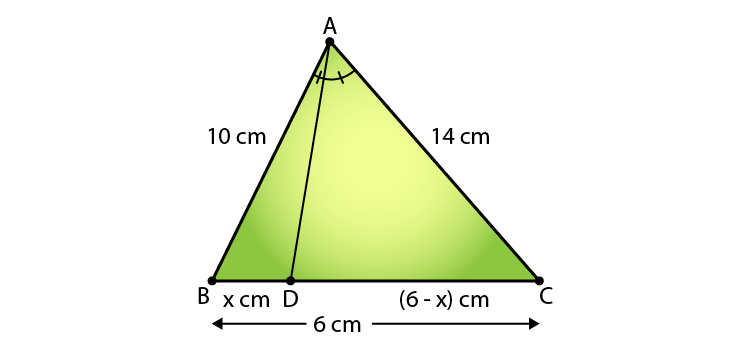Guru

# In a ∆ABC, AD is the bisector of ∠A, meeting side BC at D. If AB = 10 cm, AC = 14 cm and BC = 6 cm, find BD and DC.

• 0

Question extracted from RD sharma of class 10th, Chapter no. 4
Chapter name:- Triangles
Exercise :- 4.3
This is very basic and important questions. And has been asked in several times in exams.

In this question it is given that In a ∆ABC, AD is the bisector of ∠A, meeting side BC at D.

Also it is given that AB = 10 cm, AC = 14 cm and BC = 6 cm,

Now we have to find  the length of BD and DC.

learning CBSE maths in effective way.
RD sharma, DHANPAT RAI publication

Share

1. Given: In Δ ABC, AD is the bisector of ∠A meeting side BC at D. And, AB = 10 cm, AC = 14 cm, and BC = 6 cm

Required to find: BD and DC.Since, AD is bisector of ∠A

We have,

AB/AC = BD/DC (AD is bisector of ∠ A and side BC)

Then, 10/ 14 = x/ (6 – x)

14x = 60 – 6x

20x = 60

x = 60/20

∴ BD = 3 cm and DC = (6 – 3) = 3 cm.

• 0